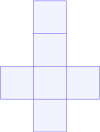# Cube

Go to Surface Area or Volume

Cube Facts

Notice these interesting things:

• It has 6 Faces
• Each face has 4 edges (and is a square)
• It has 12 Edges
• It has 8 Vertices (corner points)
• and at each vertex 3 edges meet
• It is one of the Platonic SolidsA cube is also a hexahedron because it is a polyhedron with 6 (hexa- means 6) faces.Cubes make nice 6-sided dice, because they are regular in shape, and each face is the same size.

In fact, you can make fair dice using all of the Platonic Solids.

 Make your own Cube, cut out the shape and glue it together.Salt crystals grow in cube-like shapes.
(Because the atoms link up as corners of a cube.)

## Surface Area

Surface Area = 6 × (Edge Length)2

### Example: Edge Length = 4

Surface Area= 6 × (Edge Length)2
= 6 × 42
= 6 × 16
= 96

## Volume

Volume = (Edge Length)3

### Example: Edge Length = 4

Volume= (Edge Length)3
= 43
= 4 × 4 × 4
= 64